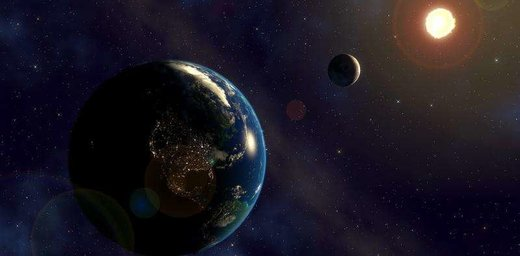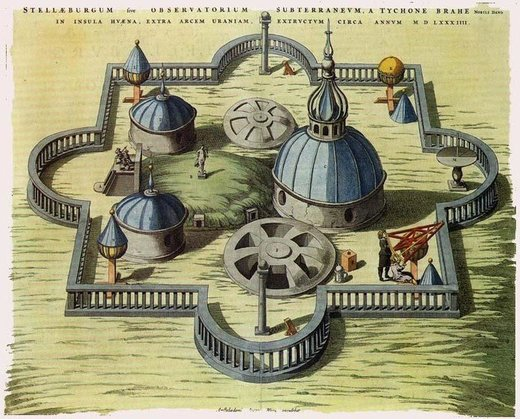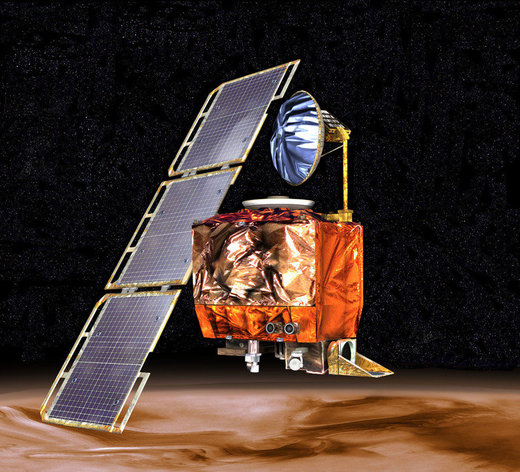Modelling three bodies interacting with each other is harder than it may seem.
From the transforming discovery of penicillin to the theories of relativity and quantum mechanics, science progressed with mind-boggling speed even before there were computers. Much of this is down to the robustness of the scientific method: scientific results are validated by being replicated and extended by other scientists.

But the way we do science is changing - we now rely increasingly on complex computer models to understand nature. And it turns out that these models can be nearly impossible to reproduce - meaning an important touchstone of science is being challenged. So what are the real-world repercussions of this change and what can we do about it?

Pre-modern science - known as "natural philosophy" - was empirical. Empirical science uses past observations to make predictions about the future, which may then be tested. Tycho Brahe, a 16th-century Danish astronomer, managed to make accurate and comprehensive observations of the heavens in this way.Drawing of Tycho Brahe’s observatory in Denmark.
Modern science, however, is theoretical. Theoretical science also makes predictions, but it derives them from mathematical models rather than from prior observations. Think of Isaac Newton's laws of motion, such as the inverse square law of gravitation.

Such mathematical equations are great when they are available - but often they are not. For example, we know that there is no simple equation that solves the so-called "three-body problem", which describes three bodies orbiting around and influencing each other by gravitational forces - like the moon, Earth and sun.

Much of current science deals with even more complicated systems, and similarly lacks exact solutions. Such models have to be "computational" - describing how a system changes from one instant to the next. But there is no way to determine the exact state at some time in the future other than by "simulating" its evolution in this way. Weather forecasting is a familiar example; until the advent of computers in the 1950s, it was impossible to predict future weather faster than it actually happened.

Current science typically consists of devising a mathematical model that describes a complicated system, then turning this into a computational simulation, and running the simulation to make predictions in order to validate the model.

When modelling fails

Modelling is used across scientific fields - ranging from astrophysics and climate prediction to bioinformatics and economics. But there is increasing debate about the fact that this science is difficult to validate through reproduction.

It turns out that simply describing experimental methods in words is not enough. That's partly because natural languages such as English are simply too vague for describing computations precisely. There is, after all, a reason why programmers use programming languages. One of the biggest challenges in software development is in converting vague requirements into precise specifications of behaviour.

Humans - even scientists - are after all fallible. Transforming any information into a program almost invariably introduces bugs along the way. For example, many scientists depend on data exploration tools such as spreadsheets, which are designed for ease of use and not for robustness. It is very easy simply to sum up the wrong range of cells in a spreadsheet, without getting any warnings. This was one of the methodological flaws in a paper that the US Republican Party used to base their pro-austerity policies on.

Similarly a recent study on the 15,770 spreadsheets that were made public during the investigation into the US corporation Enron showed that 24% of the spreadsheets containing at least one formula had obvious bugs, such as adding up blank cells.